Main Page - Back

# Unique Rectangles

From sudokuwiki.org, the puzzle solver's siteUnique Rectangles takes advantage of the fact that published Sudokus have only one solution. If your Sudoku source does not guarantee this then this strategy will not work. But it is very powerful and there are quite a few interesting variants.

Credits first: The ideas for these strategies I have lifted wholesale from MadOverLord's description (11 Jun 05) on the forum at www.sudoku.com. (Sadly the thread is no longer hosted). To my credit I have provided my own examples. (please email me for other credit requests). I will stick to MadOverLord's nomenclature.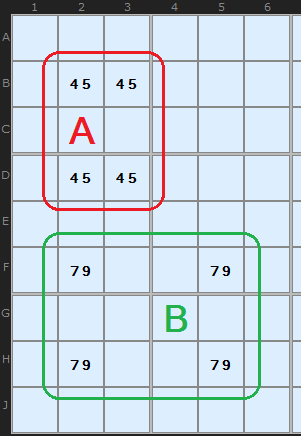The 'Deadly Pattern' In Figure 1 we have two example rectangles formed by four cells each. The pattern in red marked A consists of four conjugate pairs of 4/5. They reside on two rows, two columns and two boxes. Such a group of four pairs is impossible in a Sudoku with one solution. The reason? Pick any cell with 4/5. If the cell solution was 4 then we quickly know what the other three cells are. But it would be equally possible to have 5 in that cell and the others would be the reverse. There are two solutions to any Sudoku with this deadly pattern. If you have achieved this state in your solution something has gone wrong.

The pattern ringed in green looks like a deadly pattern but there is a crucial difference. The 7/9 still resides on two rows and two columns, but instead of two boxes it is spread over four boxes. Now, such a situation is fine since you can't guarantee that swapping the 7 and 9 in an alternate manner will produce two valid Sudokus. One of them is the real solution, the other a mess. Why? Swapping the 7 and 9 around places them in different boxes and 1 to 9 must exist in each box only once. In the red example, swapping within the box does not change the content of that box.

## Type 1 Unique Rectangles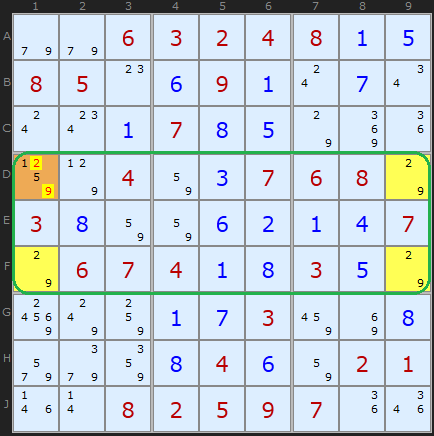Unique Rectangle Figure 2 : Load Example or : From the Start For all Unique Rectangles we are going to look for potential deadly patterns and take advantage of them. A Type 1 Unique Rectangle is illustrated in Figure 2. The three yellow cells marked contain 2/9. The fourth corner marked in orange also contains 2/9 and two other candidates. If the 1/5 were removed from that cell we would have a Deadly Pattern. This cannot be allowed to happen so its safe to remove 2 and 9 from that cell.

The proof is pretty straightforward once you get your head around the basic idea. Assume D1 is 2. That forces D9 to be 9, F9 to be 2, and F1 to be 9. That's the deadly pattern; you can swap the 2's and 9's and the puzzle still can be filled in. So if the Sudoku is valid, D1 cannot be 2. The exact same logic applies if you assume D1 is 9.
So D1 can't be a 2, and can't be a 9
- it must be either 1 or 5.

## Type 2 Unique Rectangles

In Figure 3 we have a similar pattern, but this time, A5 and A6 (cells which are also in the same box) both have a single extra possibility - in this case, 7.

To make subsequent discussion easier to follow, we will refer to the two squares that only have two possibilities as the floor squares (because they form the foundation of the Unique Rectangle); the other two squares, with extra possibilities shall be called the roof squares.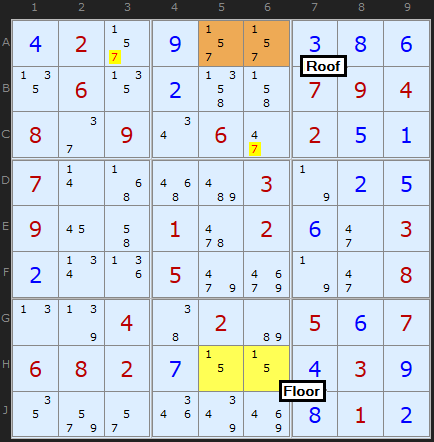Unique Rectangle Figure 3 : Load Example or : From the Start In this "Type-2 Unique Rectangle", one of the boxes contains the floor squares, and the other contains the roof squares. In order to avoid the deadly pattern, 7 must appear in either A5 or A6 (the roof squares). Therefore, it can be removed from all other cells in the units (row, column and box) that contain both of the roof cells (in this case, row 1 and box 2).

Now that you've gotten your head around the basic unique rectangle concept, the proof should come clear:

If neither A5 or A6 contains an 7, then they both become cells with possibilities 1/5. This results in the deadly pattern - so one of those cells must be the 7, and none of the other squares in the intersecting units can contain 7. So A3 and C6 can have 7 removed. This cracks the Sudoku.
I couldn't resist adding this example which I found while looking for Empty Rectangles. It's as clear as day how the 8s in H4 and H6 combine with the [1,6] Deadly Pattern. 8's aligned with the brown cells are eliminated. I need say no more.

## Type 2B Unique Rectangles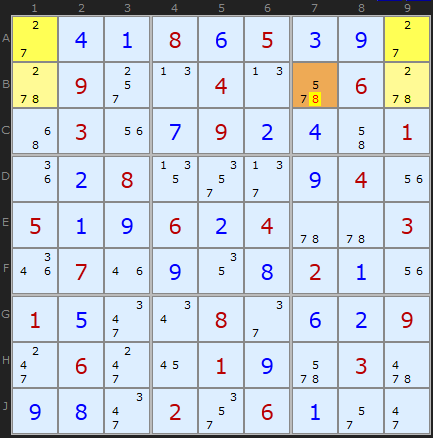Unique Rectangle Figure 5 : Load Example or : From the Start There is a second variant of Type-2 Unique Rectangles as illustrated In Figure 5.

In this puzzle, we have the same pattern of 4 squares in 2 boxes, 2 rows and 2 columns. The floor squares are A1 and A9, and the roof squares are B1 and B9. However, in this Unique Rectangle, each of the boxes contains one floor and one roof cell. This is perfectly fine, but it means that the only unit (row/column/box) that contains both of the roof cells is row 2, so that is the only unit that you can attempt to reduce; in this case, B7 cannot contain a 8. This is called at "Type-2B Unique Rectangle".

You will need to untick 3D-Medusa to see this example.

## Type 2C Unique RectanglesUnique Rectangle Figure 6 : Load Example or : From the Start HoDoKu records this as a Type 5 but since it's a variation on Type 2 I'm going to categorize it as 2C. It is fairly rare but easy to understand.

This variation again has the extra candidate but in cells diagonally opposite. You can see the extra 6 in cells B7 and H9 (marked as C and D). 6 Must exist in one of those two cells so any 6 not in the deadly rectangle pattern that can 'see' C and D can be removed.

Not shown in the example is the chance that A or B can also contain the extra candidate 6. Lets say B did - the eliminations hold since those cells can see B9. But if the third extra candidate was in H7 then we could not look in box 3.

## Type 3 Unique Rectangles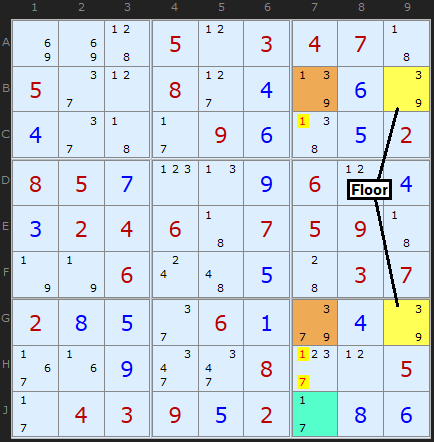Unique Rectangle Figure 7 : Load Example or : From the Start In this variant we have a floor with a pair as before but the roof contains two different candidates (occurring once or twice in each cell of the roof). In the Figure 7 the floor and roof contain [3/9] and the extra candidates in the roof are 1 and 7. Removing both the 1 and 7 from the roof would leave the deadly pattern so either the 1 in B7 must be a solution or the 7 in G7 is a solution. Knowing this does not get us as far as an elimination, however, but we can say that the 1 in B7 and the 7 in G7 act as a pseudo-cell in their own right. The clever bit is using this pseudo-cell with a bi-value cell containing the same candidates. Such a cell is circled on the board � J7. We effectively have a �locked set� like a Naked Pair. 1 will occur in B7 or J7 or 7 will occur in G7 or J7, we just don�t know which way round.

Such reasoning allows us to remove other 1s and 7s on the unit shared by the roof cells (but not the cell we�re using to create a locked set with). There are 1s and 7s in C7 and H7 which can be eliminated.

## Type 3b Unique Rectangles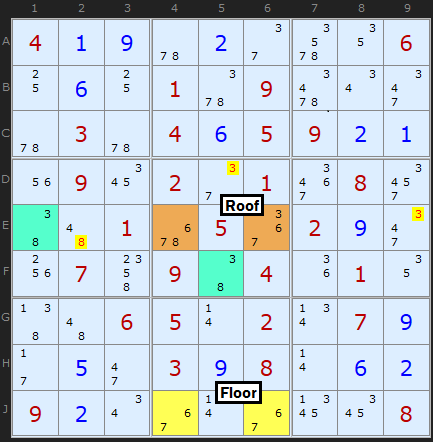Unique Rectangle Figure 8 : Load Example or : From the Start There is a compliment to Type 3, where the roof cells share the same box and that means the floor must be in a different box. Cells J4 and J6 form a pair with 6 and 7. These can see two cells in row E which also contain 6/7 as well as a 3 and an 8. We need to match this 3 and 8 in the roof cells with a bi-value cell to make a locked set (effectively a Hidden Pair). As we have roof cells in the same row AND box we can look in both row 5 to E1 and remove all other 3s and 8s in the row, plus F5 and eliminate 3 from D5.

I'd like to credit Herv� G�rard for the first example which he sent to me in November 2013 and later Tom Morrin for stating the box should be used as well.

## Type 3/3b with Triple Pseudo-Cells

So far Type 3 and 3b have worked with a pseudo-cell linking with a bi-value cell to make a pseudo pair. This is a valid locked set. Well, locked sets don't have to be pairs, they can involve three candidates over three cells.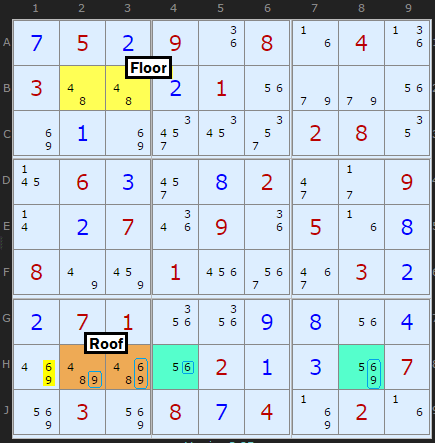Type 3b with a Triple : Load Example or : From the Start In this example we have a deadly rectangle based on 4 and 8. The remaining candidates in the Roof are 6 and 9. Whether there are one or two of either 6 and 9 in the Roof is not important. We know 6 OR 9 has to appear in H23. Members of a Locked Set must all see each other, so we are looking at other cells that align with the roof, namely row H (and occasionally the box as well). For a Triple cell Locked Set we need two other cells that contain 6 and 9 and one other candidate not present in the Roof. H4 contains 5 and 6 and H8 5, 6 and 9. With our pseudo-cell that makes a Naked Triple. Naked Triple rules apply so 6 and 9 can be removed from H1.

All credit to David Hollenberg for working this insight that nicely expands the strategy. In a test of 5,000 puzzles with some kind of UR, a third more Type 3 and 3B were found with Triples.

## Type 4 Unique Rectangles

- Cracking the Rectangle with Conjugate Pairs

An interesting observation is that it is sometimes possible to remove one of the original pair of possibilities from the roof squares. Consider the following puzzle in Figure 10 which is a continuation of the Sudoku puzzle used in the first example.

Look closely at the roof squares, H1 and H2, but this time, don't look at their extra possibilities; look at the possibilities they share with the floor squares.

If you look carefully, you'll see that in box 7, the roof squares are the only squares that can contain a 7. This means that, no matter what, one of those squares must be 7 - and from this you can conclude that neither of the squares can contain a 9, since this would create the "deadly pattern"! So you can remove 9 from H1 and H2.

Nomenclature: When two squares are the only two squares in a unit that can have a particular value, they are referred to as a conjugate pair on that value.

This is an example of a "Type-4 Unique Rectangle". As you have probably realised, since the roof squares are in the same box, you can search for conjugate pairs in both of their common units (the row and the box, in this case).

## Type 4B Unique Rectangles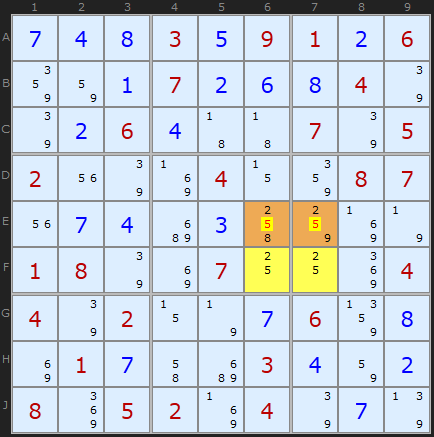Unique Rectangle Figure 11 : Load Example or : From the Start And, as you might expect, there is a Type-4B Unique Rectangle variant, in which the floor squares are not in the same box, and you can only look for the conjugate pair in their common row or column. For example:

In this case, since 2 can only appear in row E in the roof squares, 5 can be removed from both of them.

As Type-4 Unique Rectangle solutions "destroy" the Unique Rectangle, it is usually best to look for them only after you've done any other possible Unique Rectangle reductions.